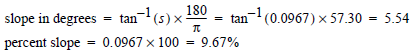# Slope Images

## Producer Field Guide

HGD_Product
Producer Field Guide
HGD_Portfolio_Suite
Producer

Slope is expressed as the change in elevation over a certain distance. In this case, the certain distance is the size of the pixel. Slope is most often expressed as a percentage, but can also be calculated in degrees.

Use Slope function to generate a slope image.

In ERDAS IMAGINE, the relationship between percentage and degree expressions of slope is as follows:

• a 45° angle is considered a 100% slope
• a 90° angle is considered a 200% slope
• slopes less than 45° fall within the 1 - 100% range
• slopes between 45° and 90° are expressed as 100 - 200% slopes

A 3 × 3 pixel window is used to calculate the slope at each pixel. For a pixel at location X,Y, the elevations around it are used to calculate the slope as shown in the figure below. In this figure, Pixel X,Y has elevation value e. The pixels around Pixel X,Y in a 3 x 3 window have the elevations a, b, c, d, e, f, g, h, and i. Each pixel has a ground resolution of 30 × 30 meters.

3 × 3 Window Calculates the Slope at Each Pixel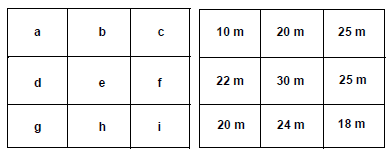First, the average elevation changes per unit of distance in the x and y direction (Dx and Dy) are calculated as: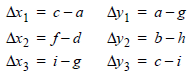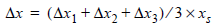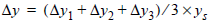Where:

a...i = elevation values of pixels in a 3 × 3 window, as shown above

xs = x pixel size = 30 meters

ys = y pixel size = 30 meters

The slope at pixel x,y is calculated as: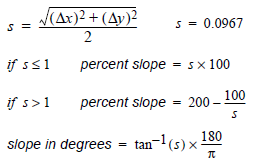Example

Slope images are often used in road planning. For example, if the Department of Transportation specifies a maximum of 15% slope on any road, it would be possible to recode all slope values that are greater than 15% as unsuitable for road building.

A hypothetical example is given in the figure below, showing how the slope is calculated for a single pixel. The shaded pixel is the pixel for which slope is being calculated. The elevations of neighboring pixels are expressed in meters.

Slope Calculation Example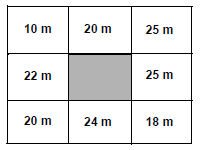So, for the hypothetical example: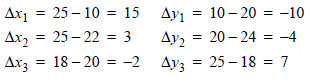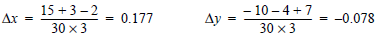For the example, the slope is: